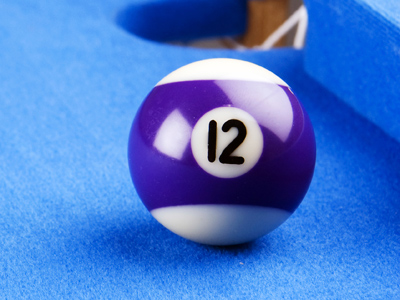Rapid Revision For School StudentsAnother name for 12 is a dozen.

# 2 Times Table

In KS1 Maths and Numeracy children will be exposed to the times tables as early as possible in Year 1. The 1, 2, 5 and 10 times tables are taught first before the 3, 4, 6, 7, 8 and 9 times tables follow. By the end of Year 2 children are often fluent in their times tables up to 10.

This quiz will test children's familiarity with their two times table, which will help them in studying maths in Years 1 and 2.

Do you know your two times table? To multiply a number by two you just double it.

1.
6 x 2 =
12
10
13
18
12 is also called a dozen
2.
10 x 2 =
15
5
12
20
To multiply a number by 10 just put a 0 on the end of it!
3.
2 x 4 =
6
8
7
10
4.
2 x 5 =
20
15
10
5
5.
9 x 2 =
16
18
20
17
9 x 2 is the same as 9 + 9. Both come to 18
6.
2 x 2 =
6
1
4
2
7.
1 x 2 =
0
1
2
4
Any number multiplied by 1 gives the same number, so 1 x 1 = 1, 2 x 1 = 2, 3 x 1 = 3 etc.
8.
2 x 7 =
12
16
15
14
9.
2 x 3 =
6
4
2
3
10.
8 x 2 =
16
14
18
20
8 + 8 = 16, so 8 x 2 = 16
Author:  Graeme Haw